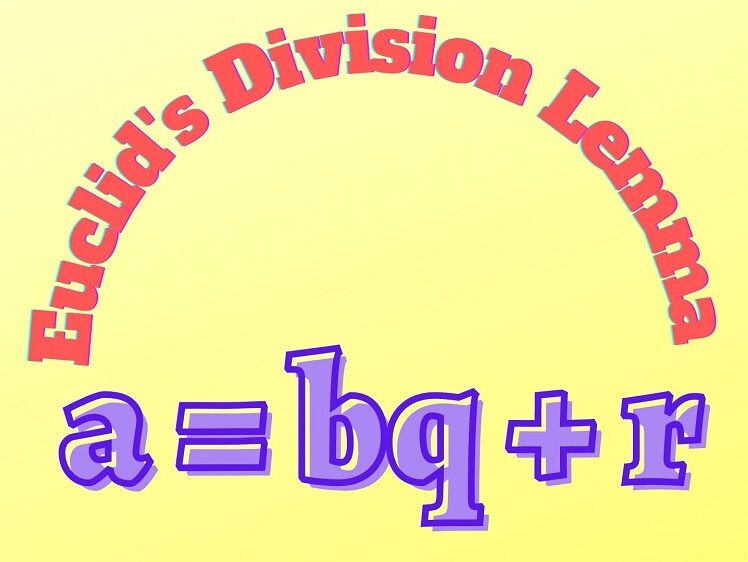## Euclid’s Division Lemma Class 10th

Introduction Euclid’s Division Lemma is generally an algorithm that is derived by Greek Mathematician Euclid. This lemma is based on the Division of Real Numbers. We have already studied the division algorithm, in which if we divide a number by another number we get two numbers a quotient and a remainder (sometimes the remainder may …